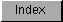```
The blitter can combine the data from the three source  DMA channels  in
up to 256 different ways to generate the values stored by the destination
DMA channel . These sources might be one bitplane from each of three
separate graphics images.  While each of these sources is a rectangular
region composed of many points, the same logic operation will be performed
on each point throughout the rectangular region.  Thus, for purposes of
defining the blitter logic operation it is only necessary to consider what
happens for all of the possible combinations of one bit from each of the
three sources.

There are eight possible combinations of values of the three bits, for
each of which we need to specify the corresponding destination bit as a
zero or one. This can be visualized with a standard truth table, as shown
below. We have listed the three source channels, and the possible values
for a single bit from each one.

A       B       C       D  BLTCON0  position   Minterm
-       -       -       - ------------------ ---------
___
0       0       0       ?         0            ABC
__
0       0       1       ?         1            ABC
_ _
0       1       0       ?         2            ABC
_
0       1       1       ?         3            ABC
__
1       0       0       ?         4            ABC
_
1       0       1       ?         5            ABC
_
1       1       0       ?         6            ABC

1       1       1       ?         7            ABC

This information is collected in a standard format, the LF (Logic
Function) control byte in the  BLTCON0  register. This byte programs the
blitter to perform one of the 256 possible logic operations on three
sources for a given blit.

To calculate the LF control byte in  BLTCON0 , fill in the truth table
with desired values for D, and read the function value from the bottom of
the table up.

For example, if we wanted to set all bits in the destination where the
corresponding A source bit is 1 or the corresponding B source bit is 1, we
would fill in the last four entries of the truth table with 1 (because the
A bit is set) and the third, fourth, seven, and eight entries with 1
(because the B bit is set), and all others (the first and second) with 0,
because neither A nor B is set.  Then, we read the truth table from the
bottom up, reading 11111100, or \$FC.

For another example, an LF control byte of \$80 ( = 1000 0000 binary) turns
on bits only for those points of the D destination rectangle where the
corresponding bits of A, B, and C sources were all on (ABC = 1, bit 7 of
LF on). All other points in the rectangle, which correspond to other
combinations for A, B, and C, will be 0. This is because bits 6 through 0
of the LF control byte, which specify the D output for these situations,
are set to 0.

Designing the LF Control Byte with Minterms
Designing the LF Control Byte with Venn Diagrams

```